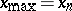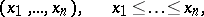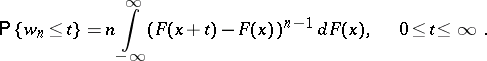# Range (of variation of a sample)

(diff) ← Older revision | Latest revision (diff) | Newer revision → (diff)

The differencebetween the largestand smallestvalues in the ordered sampleobtained by takingindependent measurements of the same random variable. Letbe the distribution function of the random variable. Then the probability distribution for the range isHow to Cite This Entry:
Range (of variation of a sample). Encyclopedia of Mathematics. URL: http://encyclopediaofmath.org/index.php?title=Range_(of_variation_of_a_sample)&oldid=14775
This article was adapted from an original article by T.Yu. Popova (originator), which appeared in Encyclopedia of Mathematics - ISBN 1402006098. See original article